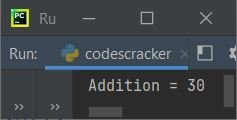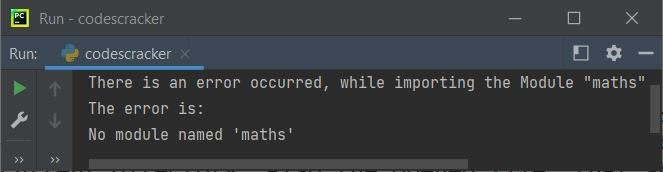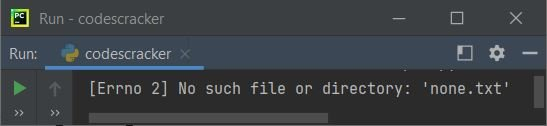# Python as Keyword

The as keyword in Python is used to provide aliases. Basically as keyword is used, most of the time to define a sub-name or user-defined name for any pre-defined name. For example:

`import operator as op`

Now the module operator is accessed using its duplicate name say op. That is, whenever in the program, you type op, means it represents to operator module. For example:

```import operator as op

a = 10
b = 20

As you can see, the op variable referred to operator, therefore using the function add() of operator module, the addition of two numbers gets performed using op.add(). That is exactly equal to operator.add(). Here is the output produced by above program:## Contexts Where as Keyword Can Be Used

Here are the list of four contexts where the keyword as can be used to give aliases, normally.

1. as keyword to give aliases for modules
2. as keyword to give aliases for sub-modules
3. as keyword to give aliases for resources
4. as keyword to give aliases for exceptions

Let's briefly explain these contexts along with example programs.

### as Keyword To Give Alias For Module

The as keyword, most of the time, used to give alias or sub-name to the module. The program given below demonstrates this context.

`import <module_name> as <alias_name>`

For example, the program given below, gives the alias say r to random module.

```import random as r

print(r.randint(10, 100))```

produces a random number between 10 and 100. As you can see from the above program, the variable r after the statement:

`import random as r`

is considered as random. Therefore, wherever we write r, means, we're writing random after the above statement.

### as Keyword To Give Alias For Sub Module

The as keyword can also be used to give alias to the sub module. Here is the syntax shows, how to use as keyword to give alias for sub module in Python:

`from <module_name> import <sub_module_name> as <alias_name>`

For example:

```from math import sqrt as s

print(s(25))```

In above program, the s inside the print() function, is basically sqrt function of math module. Here is the output, you'll see after executing the above code:

`5.0`

### as Keyword To Give Alias For Resource

The as keyword along with with keyword, can be used to provide alias for the resource in a Python program. Let's see the syntax first, then will see the example:

`with <resource> as <alias_name>`

For example, the following program, creates a file named codescracker.txt and writes Hello there! to this file. If the file codescracker.txt is already available in the current directory, then the program doesn't creates the file, only overwrites the content with Hello there!. Here is the program:

```with open('codescracker.txt', mode='w') as fh:
print('Hello there!', file=fh)```

After executing the above program, a file named codescracker.txt is created (if not available previously) with given content, that is Hello there! as shown in the snapshot given below. The snapshot shows both, that is, the file available in current directory and the opened file shows the written/overwritten content using the above program:Note - If you're confusing on, how the print() statement writes content to file, instead of writing to output screen. Then refer to its separate tutorial.

The above program, can also be created in this way:

```with open("codescracker.txt", "w") as fh:
fh.write("Hello there!")```

The actual task of this and the previous program, is exactly same.

### as Keyword To Give Alias For Exception

This is the last context, where the as keyword can be used. To produce the default exception raised by any exception name, use as keyword to give the alias for the exception, and print the default exception error as shown in the program given below. But let's see the syntax first:

`except <exception_name> as <alias_name>`

For example:

```try:
import maths as m
print("The \"maths\" module is imported with \"m\" as its Duplicate Name.")
except ImportError as ie:
print("There is an error occurred, while importing the Module \"maths\"")
print("The error is:")
print(ie)```

Since there is no any module named maths available in Python. Therefore, here is the output produced by above program:Note - The math module is available in Python, not maths.

Let's create another program, uses as keyword to give alias for the exception name say FileNotFoundError, in this case:

```try:
with open("none.txt", "r") as fh:
except FileNotFoundError as fnfe:
print(fnfe)```

Since the file none.txt is not available in the current directory. Also the file is opened using r, that is reading mode. Therefore, if the file is found to be not available in the current directory, does not creates a new file, rather produces or raises an error. Therefore, here is the output produced by above program:Python Online Test

« Previous Tutorial Next Tutorial »